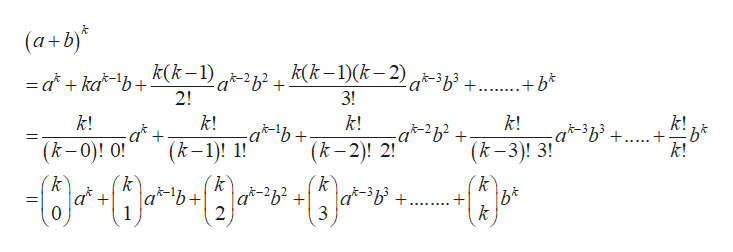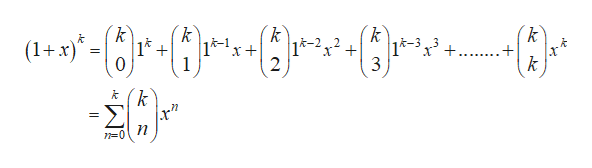Write the power series for (1 + x)k in terms of binomial coefficients.

Question

Write the power series for

(1 + x)k

in terms of binomial coefficients.

Step 1

The binomial theorem is given below:help_outlineImage Transcriptionclose(a+b)* =d+ka*\b+ k(k-1) q-2z2 + k(k -1)(k,2) q-3z3 + +* 2! 3! k! k! -ab k! k! k! +... k! (k-3)! 3! (k-1)! (k-2)! 2! (k-0)! 0! k C} k k k a-2b2 + a*-3b3 +. k 2 3 fullscreen
Step 2

We need to write the power series for (1 + x)k   in terms of...help_outlineImage Transcriptionclosek k k (1x) 14 k-1 1-212 2 |k-3 3 + 0 1 3 k fullscreen

Want to see the full answer?

See Solution

Want to see this answer and more?

Our solutions are written by experts, many with advanced degrees, and available 24/7

See Solution
Tagged in

Calculus